﻿ From order to chaos - Problems and lessons - Education - Saratov group of theoretical nonlinear dynamics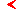main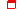this page only

# Computer practical lessons for course"From order to chaos"educationprogram

## Topic "Fractal properties of the critical attractor at the onset of chaos via period-doubling cascade"

1. Compose a program to locate unstable cycles of period 2, 4, 8,...2k for logistic map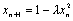at the critical point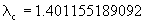and calculate multipliers (stability eigenvalue) for these cycles (up to period 4096).

Hint: Use a well convergent recurrent schema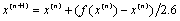, where f(x) designates a result of 2k -th iteration of the logistic map starting at x.

2. For logistic map draw several levels of the Cantor-like construction of the critical attractor on the computer screen.

3. Compose a program to draw a plot of the Feigenbaum sigma-function.

4. Calculate the Hausdorff dimension D of the critical attractor. For this, compose a program to compute sums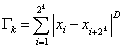and select D to make sums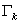and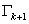equal. How does the accuracy depends on the level k?

5. Compose a program to obtain spectrum of the generalized dimensions of Rényi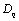and scaling-spectrum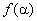. Draw plots of these functions estimated at different levels k. Compare the data with results of computations on a basis of the two-scale Cantor set model of the critical attractor. Compute as accurate as possible the information and correlation dimensions of the critical attractor.

## Topic "Noise effect onto period-doubling systems"

1. Depict on computer screen a bifurcation tree and Lyapunov exponent plot for logistic map with additive noise: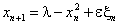, where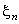is a random sequence generated by computer (with zero mean). Demonstrate that the noise destroys subtle structure of the bifurcation tree with increasing power as we consider higher levels of its organization. How does the noise influence onto position of the border of chaos?

2. Demonstrate scaling on the bifurcation tree and on the Lyapunov exponent plot. For this redraw the plot several times with subsequent rescaling for x by factor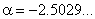, for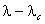by factor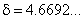(here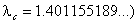, for Lyapunov exponent by factor 2, and for the noise intensity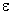by factor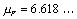(the last constant has been found first by Crutchfield et al.).

3. Invent a way to utilize the scaling property to estimate the noise scaling constant for mappings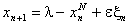with extremum of order N=4, 6, and 8.

4. Draw the charts of Lyapunov exponents for cubic map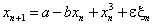at different values of the noise amplitude. How is the chaos border transformed and how is changed location of regimes of maximal stability?maineducationthis page only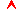top

Saratov group
of theoretical nonlinear
dynamics